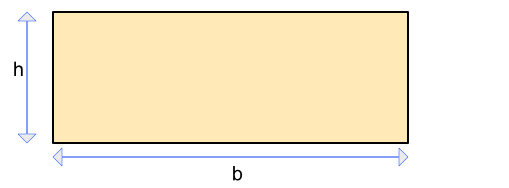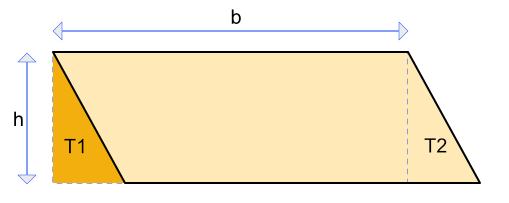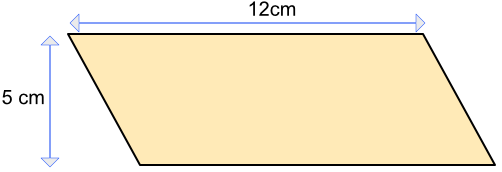Area of a Parallelogram

# Area of a Parallelogram

GCSE(F),

The area of a parallelogram is given by base x vertical height.

This can be deduced from the area of a rectangle, which is given by A = bhMove a triangle from one end of the rectangle (T1), and position on the other end (at T2). The area of the parallelogram is the same as the area of the rectangle.Note that it is the vertical distance that provides the height.

## Examples

1. What is the area of the parallelogram?Area of a parallelogram is base x vertical height

A = 12 x 5

A = 60

2. A parallelogram has an area of 100 cm2. The vertical height is 5 cm. What is the base length of the parallelogram?